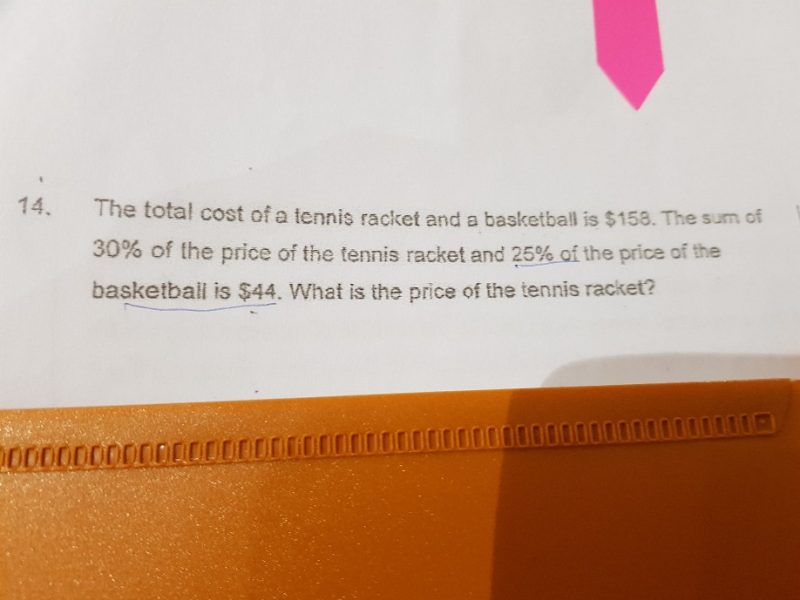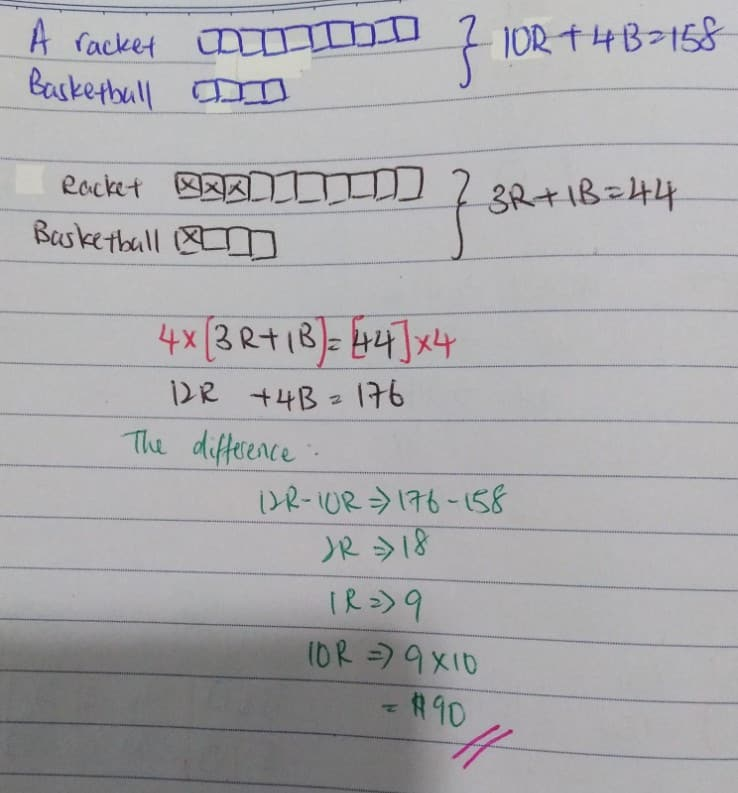# Question1 tennis racket + 1 basketball ——-> 158
25 tennis rackets + 25 basketballs ——-> 25 x 158 = 2950
0.3 tennis racket + 0.25 basketball ——-> 44
30 tennis rackets + 25 basketballs ——-> 4400
30 – 25 = 5
5 tennis rackets ——-> 4400 – 2950 = 450
1 tennis rackets ——-> 450/4 = 90

Ans : \$90.

0 Replies 0 Likes

30% = 3/10     and      25% = 1/4

Tennis Racket –> 10T    and      Basketball –> 4B

10T + 4B = \$158

3T + 1B = \$44

12T + 4B = \$176 (4 times of 3T + 1B)

2T = \$176 – \$158 = \$18

1T = \$18 ÷ 2 = \$9

10T = 10 x \$9 = \$90

Dear Priskhipo,

Thank you.

0 Replies 0 Likes